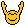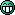# Advent of Code 2021 - Day 1

This topic is about Day 1 of the Advent of Code 2021.

We have a private leaderboard (shared with users of the elixir forum):

The entry code is:
`370884-a6a71927`

7 Likes

Here is my solution:

6 Likes

Spoiler alert! (Is it possible to mark replies as spoilers here?)

Omitting the file reading and type conversion, this is how I did it.

``````part1([A, B | T]) when B > A -> 1 + part1([B | T]);
part1([_, B | T]) -> part1([B | T]);
part1(_) -> 0.

part2([A, B, C, D | T]) when B+C+D > A+B+C -> 1 + part2([B, C, D | T]);
part2([_, B, C, D | T]) -> part2([B, C, D | T]);
part2(_) -> 0.
``````
6 Likes

I think that no spoiler markers are necessary, because it should be understood that every reply in an AoC thread is a spoiler.

6 Likes

Cool! Let code challenge begin!``````%% part1(Input, 0).
part1([H1, H2|T], Count) when H1 > H2 -> part1([H2|T], Count);
part1([_|T], Count)                   -> part1(T, Count + 1);
part1([], Count)                      -> Count - 1.

%% part2(Input, 0).
part2([H1, H2, H3, H4|T], Count) when H1 + H2 + H3 < H2 + H3 + H4 -> part2([H2, H3, H4|T], Count + 1);
part2([_|T], Count)                                               -> part2(T, Count);
part2([], Count)                                                  -> Count.
``````
5 Likes

My part 1 I used lists:foldl, having failed to do it with a list comprehension. By part 2, I realised that a recursive function was the way forward and my solution was almost identical to @vkatsuba.

5 Likes

Two videos where I try to explain my code: Advent of Code 2021 - YouTube

4 Likes

My solution is not typical from Erlang. It’s a translation from code I would write in Elixir.

I can see that most solutions use recursion``````-module(aoc1).
-export([part1/0, part2/0]).

part1() ->
{_, Sum} = lists:foldl(fun(X, {Prev, Sum}) ->
if
X > Prev -> {X, Sum + 1};
true -> {X, Sum}
end
Sum.

part2() ->
{_, Sum} = lists:foldl(fun
(X, {{nil, B, C}, Sum}) ->
{{B, C, X}, Sum};
(X, {{A, B, C}, Sum}) ->
if
B + C + X > A + B + C -> {{B, C, X}, Sum + 1};
true -> {{B, C, X}, Sum}
end
end, {{nil, nil, nil}, 0}, load_data()),
Sum.

StringContent = unicode:characters_to_list(Binary),
[element(1, string:to_integer(Substr)) || Substr <- string:tokens(StringContent, "\n")].
``````

and this is the Elixir version.

``````defmodule Aoc1 do
def part1 do
|> Enum.reduce({nil, 0}, fn x, {prev, sum} ->
if prev && x > prev, do: {x, sum + 1}, else: {x, sum}
end)
sum
end

def part2 do
|> Enum.reduce({{nil, nil, nil}, 0}, fn
x, {{nil, b, c}, sum} ->
{{b, c, x}, sum}
x, {{a, b, c}, sum} ->
if b+c+x > a+b+c,
do: {{b, c, x}, sum + 1},
else: {{b, c, x}, sum}
end)
sum
end

"input.txt"
|> String.split("\n", trim: true)
|> Enum.map(&String.to_integer(&1))
end
end
``````

I am using AOC to try to be more fluent writing Erlang, which is the opposite direction of @bjorng5 Likes

I see most of you save some time by not trying to find a good name for the functions. As I could not find good short names, I gave them names which are too long:

• depth_measurement_increases/1
• depth_measurement_increases_from_sliding_windows/1
5 Likes
``````solve_day1_1(List) ->
do_solve_day1(List, lists:nthtail(1, List)).

solve_day1_2(List) ->
do_solve_day1(List, lists:nthtail(3, List)).

do_solve_day1([LH|LT], [RH|RT]) when LH < RH ->
1 + do_solve_day1(LT, RT);
do_solve_day1([_|LT], [_|RT]) ->
do_solve_day1(LT, RT);
do_solve_day1(_, []) ->
0.
``````

The “window” is a red herring, number 1 and 2 are the same exercise with different shifts. Sadly, there is no nice way (that I know of) to make `lists:zip` behave, otherwise this could probably be golfed down quite a bit5 Likes

Yes, but you only discover that after you see Part 2. As I was doing a video, I didn’t want to change Part 1.

4 Likes

There is a small (and harmless) typo in your code, @bjorng.

Line 13: inifinity
Line 17: infinity.

4 Likes

After completing the 2017 edition and rage quitting the 2019 on video, this year I’m setting a low bar for things as I’ll try to complete as much of the advent of code as I can using only Awk, because why not.

``````#!/usr/bin/env bash

echo "part 1"
awk 'prev != "" && prev < \$1 { incr+=1; } {prev = \$1} END { print incr }' day1.input

echo "part 2"
awk \
'# initialization steps
a == "" { a=\$0; next;}
b == "" {b=a; a=\$0; next}
c == "" {c=b; b=a; a=\$0; next}
# calculate sliding window
{prev=a+b+c; c=b; b=a; a=\$0}
# count if the increment is larger
a+b+c > prev { incr+=1 }
# end result
END {print incr}' day1.input
``````
10 Likes

Awk indeed … I like your approach! Having read a couple of Haskell books but never having written any actual code, I’m using AoC as an excuse to practice. It’s good to remember that it’s okay to suck at something.

4 Likes

Nice to see many erlang solutions this yearUnfortunately I’ve got absolutely no time to participate this year4 Likes

Sounds sad. Because this is a great opportunity to practice with Erlang developers together. Some, pair programming of challenges. Do not miss the opportunity to practice with Erlang - this is a great way to learn something new and develop Erlang skills, I think that those challenges are useful not only for newcomers but also for experienced Erlang developers. But of course, up to you.

4 Likes

Yes, that’s why I did it last year already with ErlangI’d do it again this year, if I had time…
But the reason I simply have no time this year is not sad: my second daughter was born yesterdaySo I wish you all a lot of fun, while I’ll be enjoying some sleepless nights haha

10 Likes

This is amazing news! Congratulations!

4 Likes

Congrats RainerMaybe you could combine the two5 Likes

Congrats!

4 Likes